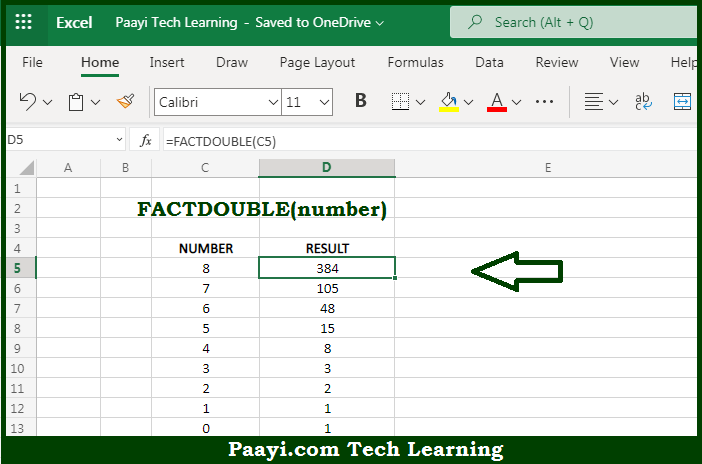# Learn How to Use Microsoft Excel FACTDOUBLE Function

Written by | 0 Comments | 508 Views

In this article, you will learn how to use the Microsoft Excel FACTDOUBLE function and its prime function in Microsoft Excel. You will also get to know the Microsoft Excel FACTDOUBLE function return value and syntax with the help of some examples.

Microsoft Excel FACTDOUBLE Function

The main function of the Microsoft Excel FACTDOUBLE function is used to find the double Factorial of a number. So, with the help of the FACTDOUBLE function, you can able to return the double Factorial of the given number. It should be noted that double factorial is symbolized by the two exclamation marks (!!). Let's say for example - =FACTDOUBLE(4), will return 576, as 4x3x2x1x4x3x2x1. So, with the help of the Microsoft Excel FACTDOUBLE function, you can easily find the double Factorial of the number.

Return Value of FACTDOUBLE Function

The return value will be the calculated double Factorial of a number.

Syntax of FACTDOUBLE Function

=FACTDOUBLE(number)

Where the arguments:

• number: This is the number of which you want to find out the double Factorial, it should be equal to or greater than -1.

## How to Use Microsoft Excel FACTDOUBLE Function?So we know that Microsoft Excel FACTDOUBLE function you can able to return the double Factorial of the given number. It should be noted that double factorial is symbolized by the two exclamation marks (!!). Let's say for example - =FACTDOUBLE(4), will return 576, as 4x3x2x1x4x3x2x1. So, with the help of the Microsoft Excel FACTDOUBLE function, you can easily find the double Factorial of the number.